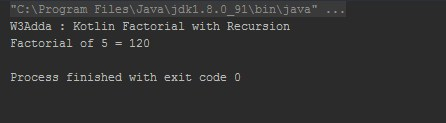# Kotlin Recursion

In this tutorial you will learn about the Kotlin Recursion and its application with practical example.

## Kotlin Recursion

Recursion is the process where a function calls itself, and the function which calls itself is know as recursive function.

## Characteristics of a recursive function

• A recursive function is a function which calls itself.
• The speed of a recursive program is slower because of stack overheads.
• A recursive function must have terminating conditions, and recursive expressions.

• It requires few variables which make program clean.
• It shorten the complex and nested code.

• It is hard to debug recursive function.
• It is tough to understand the logic of a recursive function.

Note :- Recursive function must have a valid terminating condition otherwise it leads to infinite loop.

To understand how recursion works lets have one of the popular example of recursion. In this example we will calculate the factorial of n numbers. The factorial of n numbers is expressed as a series of repetitive multiplication as shown below:

Example 1:-

Example:-

Output:-Suppose value of i=5, since i is not equal to 1, the statement:

f = i* factorial (i-1);

will be executed with i=5 i.e.

f = 5* factorial (5-1);

will be evaluated. As you can see this statement again calls factorial function with value i-1 which will return value:

4*factorial(4-1);

This recursive calling process continues until value of i is equal to 1 and when i is equal to 1 it returns 1 and execution of this function stops. We can review the series of recursive call as follow:

f = 5* factorial (5-1);

f = 5*4* factorial (4-1);

f = 5*4*3* factorial (3-1);

f = 5*4*3*2* factorial (2-1);

f = 5*4*3*2*1;

f = 120;

## Kotlin Tail Recursion

In this tutorial we have learn about the Kotlin Recursion and its application with practical example. I hope you will like this tutorial.